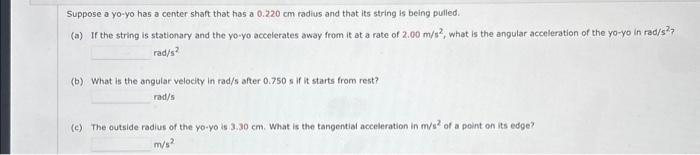# (Solved): Suppose a yo-yo has a center shaft that has a 0.220cm radius and that its string is being pulled. ...Suppose a yo-yo has a center shaft that has a radius and that its string is being pulled. (a) If the string is stationary and the yo-yo accelerates away from it at a rate of , what is the angular acceleration of the yo-yo in rad/s? (b) What is the angular velocity in rad/s after 0.750 s if it starts from rest? (c) The outside radius of the yo-yo is . What is the tangential acceleration in of a point on its edge?

We have an Answer from Expert